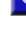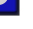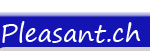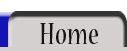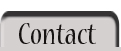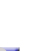All Operators / OperatorOPERATOR:   \ Implemented in version 1.0    \   The \ operator divides two numbers and returns an integer (fixed-point). Each number can be either floating-point or fixed-point.   This operator works by rounding the values of the supplied operands to integers (if necessary) before performing the calculation and only returns an integer with no decimal representation (truncated). This first example requires no rounding of the operands and returns '6' ('6.25' truncated).   Code: <% result = 25 \ 4 %>   Output: 6 This next example demonstrates the 'pre-rounding' of the operands; the actual values used for the calculation eqate to '35\6' which returns '5' ('5.833r' truncated).   Code: <% result = 35.4 \ 6.01 %>   Output: 5 Compare the previous example to the next which returns '6' because the first operand has been rounded internally to '36' before calculation.   Code: <% result = 35.9 \ 6.01 %>   Output: 6